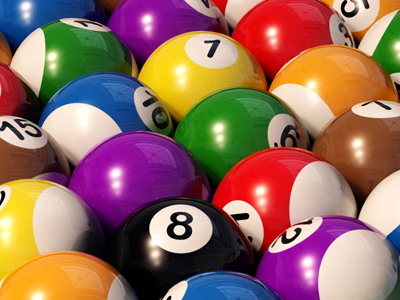How many eights in this quiz?

# Converting Decimals to Percentages

This Math quiz is called 'Converting Decimals to Percentages' and it has been written by teachers to help you if you are studying the subject at middle school. Playing educational quizzes is a fabulous way to learn if you are in the 6th, 7th or 8th grade - aged 11 to 14.

It costs only \$12.50 per month to play this quiz and over 3,500 others that help you with your school work. You can subscribe on the page at Join Us

Converting decimals to percentages means multiplying by 100.

The symbol used to show percent is “%” so 21 percent would be 21%. The “21” is the “part” and the “%” is the whole which means that 21% can also be shown as 21/100.

Converting Decimals to Percentages:

To convert a decimal to a percentage is very easy. You simply multiply the number by 100. For example, .91 would be .91 x 100 = 91. You then add the percentage symbol to make the 91 read 91%.

1.
.43 = __%
4.3%
43%
430%
4300%
To convert a decimal to a percentage you simply multiply the decimal number by 100 so .43 x 100 = 43 and then you add the percent symbol to get 43%. Answer (b) is the correct answer
2.
.35 = ___%
3.5%
.35%
.035%
35%
To convert a decimal to a percentage you simply multiply the decimal number by 100 so .35 x 100 = 35 and then you add the percent symbol to get 35%. Answer (d) is the correct answer
3.
.84 = ___%
84%
8.4%
.84%
840%
To convert a decimal to a percentage you simply multiply the decimal number by 100 so .84 x 100 = 84 and then you add the percent symbol to get 84%. Answer (a) is the correct answer
4.
.29 = ___%
2.9%
.29%
290%
29%
To convert a decimal to a percentage you simply multiply the decimal number by 100 so .29 x 100 = 29 and then you add the percent symbol to get 29%. Answer (d) is the correct answer
5.
.11 = ___%
.011%
.11%
11%
1.1%
To convert a decimal to a percentage you simply multiply the decimal number by 100 so .11 x 100 = 11 and then you add the percent symbol to get 11%. Answer (c) is the correct answer
6.
.76 = ___%
76%
.76%
7.6%
.076%
To convert a decimal to a percentage you simply multiply the decimal number by 100 so .76 x 100 = 76 and then you add the percent symbol to get 76%. Answer (a) is the correct answer
7.
.48 = ___%
40.8%
.48%
48%
4.8%
To convert a decimal to a percentage you simply multiply the decimal number by 100 so .48 x 100 = 48 and then you add the percent symbol to get 48%. Answer (c) is the correct answer
8.
.18 = ___%
18%
180%
1.8%
.018%
To convert a decimal to a percentage you simply multiply the decimal number by 100 so .18 x 100 = 18 and then you add the percent symbol to get 18%. Answer (a) is the correct answer
9.
.77 =___%
770%
7.70%
77%
.77%
To convert a decimal to a percentage you simply multiply the decimal number by 100 so .77 x 100 = 77 and then you add the percent symbol to get 77%. Answer (c) is the correct answer
10.
.90 =___%
9%
90%
900%
.9%
To convert a decimal to a percentage you simply multiply the decimal number by 100 so .90 x 100 = 90 and then you add the percent symbol to get 90%. Answer (b) is the correct answer
Author:  Christine G. Broome